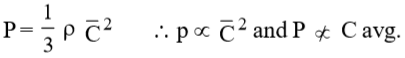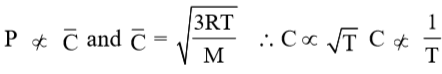According to kinetic theory of gases, for a diatomic molecule

(A) The pressure exerted by the gas is proportional to the mean velocity of the molecule.

(B) The pressure exerted by the gas is proportional to the root mean square velocity of the molecule.

(C) The root mean square velocity of the molecule is inversely proportional to the temperature.

(D) The mean translational kinetic energy of the molecule is proportional to the absolute temperature.

Concept Videos :-

#7 | Molecular Speeds & Kinetic Theory of Gases

Concept Questions :-

Kinetic Theory of Gas

(D).Difficulty Level:

• 6%
• 35%
• 16%
• 45%